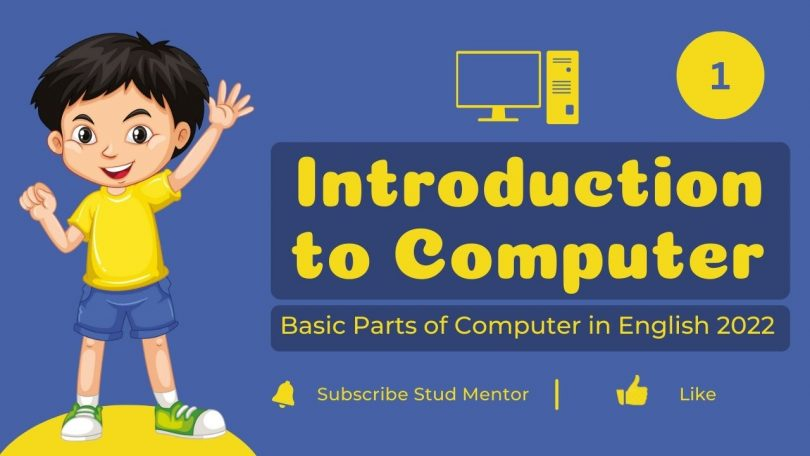# Introduction to Computer – Basic Parts of Computer in English 2022Written by

Are you searching for –  Introduction to Computer.

Then you are at Right Place.

The Complete and Official Information of Introduction to Computer – Basic Parts of Computer in English 2022.

## Introduction to Computer

Computer Literally means “to calculate” but the Modern Day computer s which are all using in life more than calculating.

Let’s understand the overview of Computer

1. Computers are Integral part of our everyday existence.
2. Literal meaning of computer is a device that can calculate.

### Definition of Computer

Computer is an electronic device that receives input, stores or processes the input as per user instructions and provides output in desired format.

Input Process Output Model

• Computer input is called Data
• Data – any raw information, raw facts, figures called Data.
• Output Obtained after processing Data, based on user’s instruction is called Information.
• Raw facts and figures which can be processed using arithmetic and logical operations to obtain information are called data.
• Processes that can be applied to data are of two types
• Arithmetic Operations
• Logical Operations

Diagram of Working Model of Computer

## Basic Parts of Computer

1. Input Unit – Devices used to input data and instructions
2. Output Unit – Devices used to provide information to the use in desired format
3. Control Unit – All devices or parts of computer interact through the control unit
4. Arithmetic Logic Unit – All Arithmetic operations and logical operations take place.
5. Memory Unit – All input data, instruction and Data Interim to the processes are stored in the memory, memory is of two types
1. Primary Memory
2. Secondary Memory